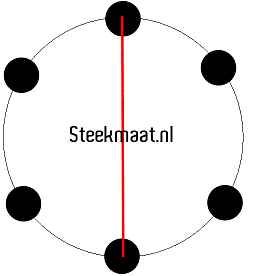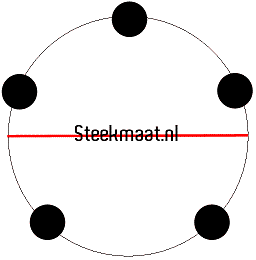Cookie Consent by FreePrivacyPolicy.com

## What is PCD?

The bolt circle is the circle determined by the positions of the bolts; the center of every bolt lies on the circumference of the bolt circle.
The important measurement is the "pitch circle diameter" (PCD), usually expressed in millimeters, although inches are sometimes used. For a 4- or 6-bolt car, this measurement is merely the distance between the center of two diametrically opposite bolts. In the 4-bolt picture this would be the distance between 2 opposite holes.Some basic geometry is needed to find the center of a 5-bolt pattern: But basically, the PCD can be found by multiplying the center distance between any two adjacent holes by 1.701.
Or just draw an imaginary circle throught the center of all holes, now measure the diameter of this circle.